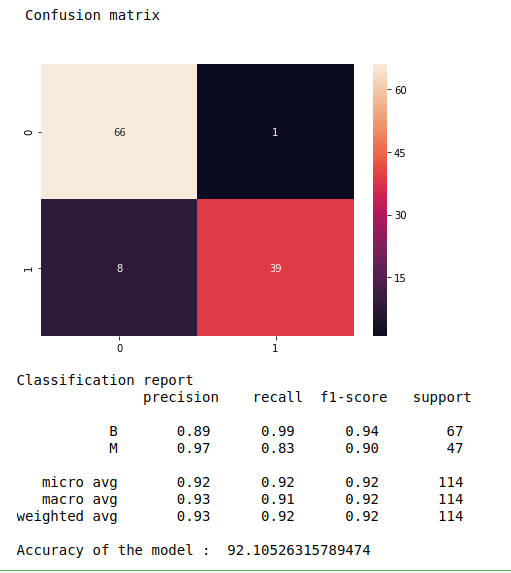• #5, First Floor, 4th Street Dr. Subbarayan Nagar Kodambakkam, Chennai-600 024 Landmark : Samiyar Madam
• pro@slogix.in
• +91- 81240 01111

### How to predict breast cancer using Multi Layer Perceptron from sklearn in python?

###### Description

To build a multi layer perceptron model for predicting breast cancer in python.

###### Input

Breast cancer. (Kaggle)

###### Output

Confusion matrix, classification report and accuracy_score.

###### Process

Import libraries.

Fix X and y variables.

Split the data into train and test.

Build the MLP model.

Fit the train data to the model.

Predict the test data.

Evaluate the model.

Print the results.

###### Sample Code

#import necessary libraries
import warnings
warnings.filterwarnings(“ignore”)
import pandas as pd
import numpy as np
import seaborn as sns
import matplotlib.pyplot as plt
from sklearn.feature_extraction.text import TfidfVectorizer
from sklearn.model_selection import train_test_split
from sklearn import metrics
from sklearn.neural_network import MLPClassifier

#make as a data frame
df = pd.DataFrame(data)

#Checking missing values
print(“Checking missing values\n”)
print(df.isnull().sum())

#Define X and y variable
X = df.iloc[:,2:32]
y = df.iloc[:,1]

#Split train and test data
X_train, X_test, y_train, y_test = train_test_split(X, y, test_size=0.2, random_state=0)

#Training and testing data
print(“\n”)
print(“After vectorized train data\n\n”,X_train,”\n\n”,y_train)
print(“After vectorized test data\n\n”,X_test)

#Neural network model

#fit the model
clf.fit(X_train, y_train)
# Predicting the Test set results
y_pred = clf.predict(X_test)
# Creating the Confusion Matrix
from sklearn.metrics import confusion_matrix
cm = confusion_matrix(y_test, y_pred)
print(“\n”,”Confusion matrix\n”)
fig, ax = plt.subplots(figsize=(7,5))
sns.heatmap(cm, annot=True, fmt=’d’)
plt.show()
print(“Classification report\n”,metrics.classification_report(y_test, y_pred))
print(“Accuracy of the model : “,metrics.accuracy_score(y_test, y_pred)*100)

###### Screenshots